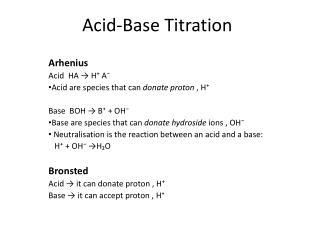DownloadDownload PresentationAcid-Base Titration

# Acid-Base Titration

Télécharger la présentation## Acid-Base Titration

- - - - - - - - - - - - - - - - - - - - - - - - - - - E N D - - - - - - - - - - - - - - - - - - - - - - - - - - -
##### Presentation Transcript

1. Acid-Base Titration Arhenius Acid HA → H⁺ A⁻ Acid are species that can donate proton , H⁺ Base BOH → B⁺ + OH⁻ Base are species that can donate hydroside ions , OH⁻ Neutralisation is the reaction between an acid and a base: H⁺ + OH⁻ →H₂O Bronsted Acid → it can donate proton , H⁺ Base → it can accept proton , H⁺

2. Choosing A Titrant In theory , any strong acid or strong base can be used as titrant. The reason for this is the most reaction involving a strong acid or strong base is QUANTITATIVE.

3. Strong And Weak Acid Titrant

4. Strong And Weak Base Titrant

5. Equivalent Point The point in the reaction at which both acid and base have been consumed. Neither acid nor base is present in excess End Point Actual result obtained by observations of sudden change of physical properties

6. End Point vs. Equivalent Point End Point • The point at which the reaction is observed to be completed • The end point signal frequently occurs at some point other than the equivalent point • Refers to point at where the indicator changes colour in the titration Equivalent Point or stoichiometric point • The point at which the equal amount of titrant is added to the analyte • Point at which the reaction is supposedly to complete

7. End Point Equivalent point

8. Strong acid with strong Base • Equivalence at pH 7 7 pH mL of Base added

9. Titration of a strong acid by a strong base Sudden sharp changes of pH Slow change of pH Titration curve for the titration of 25.00 mL of 0.2000 M HCl (a strong acid) with the 0.2000 MNaOH (a strong base).

10. Strong base with strong acid • Equivalence at pH 7 7 pH mL of acid added

11. Selecting And Using Indicators

12. ACID-BASE INDICATORS

13. Strong Acid WithStrong Base • Do the stoichiometry. • mL x M = mmol • They both dissociate completely. • The reaction is H+ + OH- HOH • Use [H+] or [OH-] to figure pH or pOH